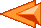## Binary handling

Let's consider the byte xxxx xBAx, where x can be either 0 or 1, and BA is a number we want to retrieve. To do so, there are 2 methods:
1. number = A + 2·B (the number is not 2a+4b since the binary number is BA and not BAx)
2. alternate method:
1. create a mask such as the bits to be retrieved are covered by "1": 0000 0110
2. do a logical AND with the byte containing the information:
 xxxx xBAx AND 0000 0110 0000 0BA0
3. right-shift this byte of 1 bit. This can be done with the >> (and respectively left-shift with <<) operator. You get: 0000 00BA
4. just read the value in the byte.## STATIC things

When modifying a variable, the static keyword specifies that the variable has static duration (it is allocated when the program begins and deallocated when the program ends) and initializes it to 0 unless another value is specified.
When modifying a variable or function at file scope, the static keyword specifies that the variable or function has internal linkage (its name is not visible from outside the file in which it is declared).

In C++, when modifying a data member in a class declaration, the static keyword specifies that one copy of the member is shared by all the instances of the class. When modifying a member function in a class declaration, the static keyword specifies that the function accesses only static members.

example:
```static int i;		// Variable accessible only from this file

static void func();		// Function accessible only from this file

int max_so_far(int curr) {
static int biggest;		// Variable whose value is retained between each function call
if( curr > biggest ) biggest = curr;
return biggest;
}

// C++ only
class SavingsAccount {
public:
static void setInterest ( float newValue )		 // Member function that accesses only static members
{
currentRate = newValue;
}
private:
char name;
float total;
static float currentRate;		// One copy of this member is shared among all instances of SavingsAccount
};

// Static data members must be initialized at file scope, even if private.
float SavingsAccount::currentRate = 0.00154;
```## The -> operator

-> is said to be equivalent to . meaning Component Selection.## Dealing with classes

A class is an evolution of a structure. A structure only contains data whereas a class contains both
• data
• functions dedicated to that class, called methods
The core of each method can be detailed directly in the class itself:
```#include <iostream.h>

class Point {
public :
int x;
int y;

void Init(int a, int b) { x = a; y = b; }
void Deplace(int a, int b) { x += a; y += b; }
void Affiche() { cout << x << ", "<< y << endl; }
};

void main() {
Point p;
p.Init(3,4);
p.Affiche();
p.Deplace(4,6);
p.Affiche();
}
```

Or outside the class (which is a better idea when the methods are complex). Please notice the special syntax: Point::
```#include <iostream.h>

class Point {
public :
int x;
int y;

void Init(int a, int b);
void Deplace(int a, int b);
void Affiche();
};

void Point::Init(int a, int b) {
x = a;
y = b;
}

void Point::Deplace(int a, int b) {
x += a;
y += b;
}

void Point::Affiche() {
cout << x << ", " << y << endl;
}

void main() {
Point p;
p.Init(3,4);
p.Affiche();
p.Deplace(4,6);
p.Affiche();
}
```## Pointers

*var
value of the variable var
&var